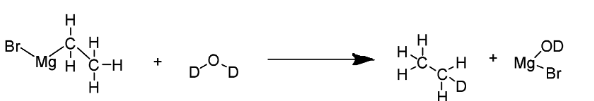# Indicate the expected structure of the organic product when ethyl magnesium bromide istreated with heavy water (${D_2}O$)$\begin{array}{*{20}{l}}{A:{C_2}{H_5} - {C_2}{H_5}} \\{B:{C_2}{H_5}OD} \\{C:{C_2}{H_6}} \\{D:{C_2}{H_5}D}\end{array}$Verified
184.2k+ views
Hint:${D_2}O$ i.e. deuterium oxide, is also known as heavy water. It is a compound made up of deuterium and oxygen.Deuterium is a heavy isotope of hydrogen, denoted by either $^2H$or $D$.
On the other hand, ethyl magnesium bromide is a Grignard reagent.Grignard reagent is basically a chemical compound having the generic formula of $R - Mg - X$, while, $X$ refers to a halogen and R refers to an organic group, primarily an alkyl or aryl group.

Complete answer:When Grignard reagent ($R - Mg - X$) is treated with water, it leads to a hydrolysis reaction yielding corresponding alkanes as well as $RMg\left( {OH} \right)X$. In this reaction, hydrogen of alkane along with hydroxyl group i.e. $OH$ in $RMg\left( {OH} \right)X$ actually comes from water i.e. ${H_2}O$.
Now in the present question, water (i.e. ${H_2}O$) is being replaced with heavy water (i.e. ${D_2}O$). Therefore correspondingly in alkane also, H will be replaced with heavy hydrogen that is $D$ and $OH$ in $RMg\left( {OH} \right)X$ will also get replaced with $OD$. Thus, the final reaction of ethyl magnesium bromide with heavy water can be written as follows:

${C_2}{H_5}MgBr + {D_2}O \to {C_2}{H_5}D + Mg\left( {OD} \right)Br$

The structure of the compounds in reaction are also depicted below:Hence, the expected structure of the organic product i.e. alkane is tetrahedral (since both carbon are sp3 hybridized) when ethyl magnesium bromide is treated with heavy water (${D_2}O$).

Therefore, the correct answer is Option D.

Note: Since deuterium’s atomic mass is greater as compared to that of protium (hydrogen), molar mass of ${D_2}O$ is more than that of ${H_2}O$. Thus, ${D_2}O$ possess slightly different physical and chemical properties in comparison to ${H_2}O$. ${D_2}O$ is non-radioactive owing to the deuterium being a stable isotope. ${D_2}O$ can also be produced via hydrogen sulfide-water chemical exchange method, electrolysis and water distillation.